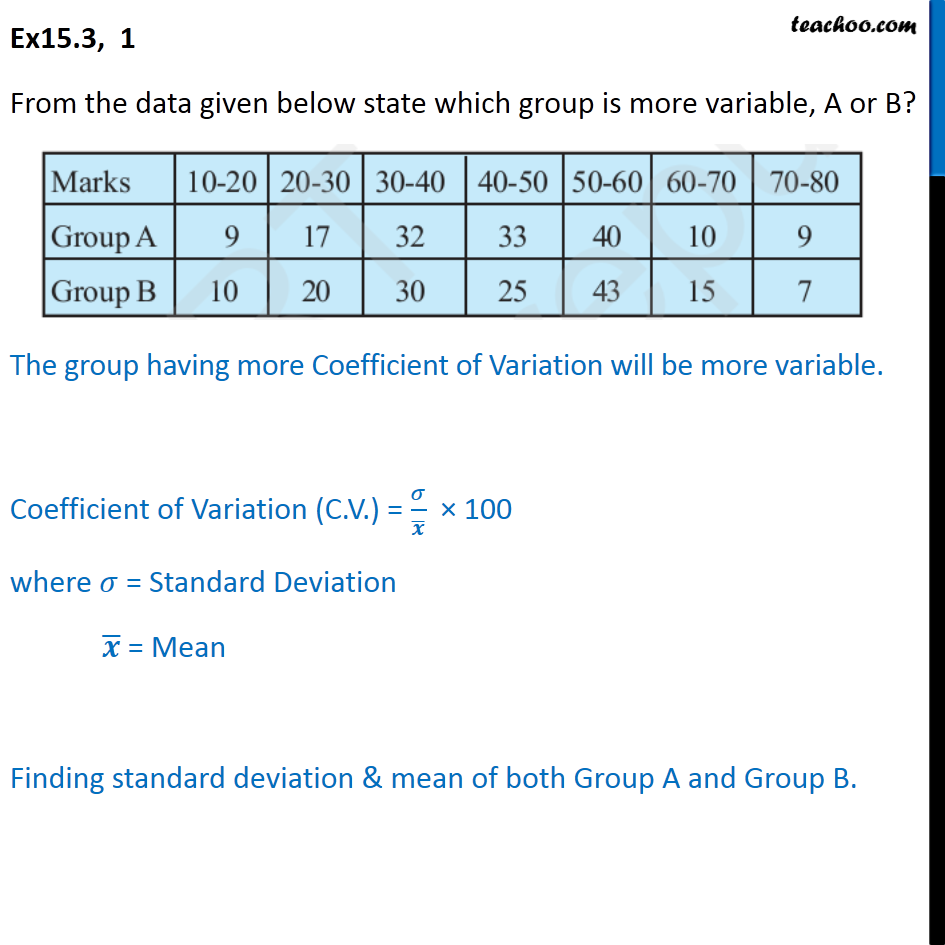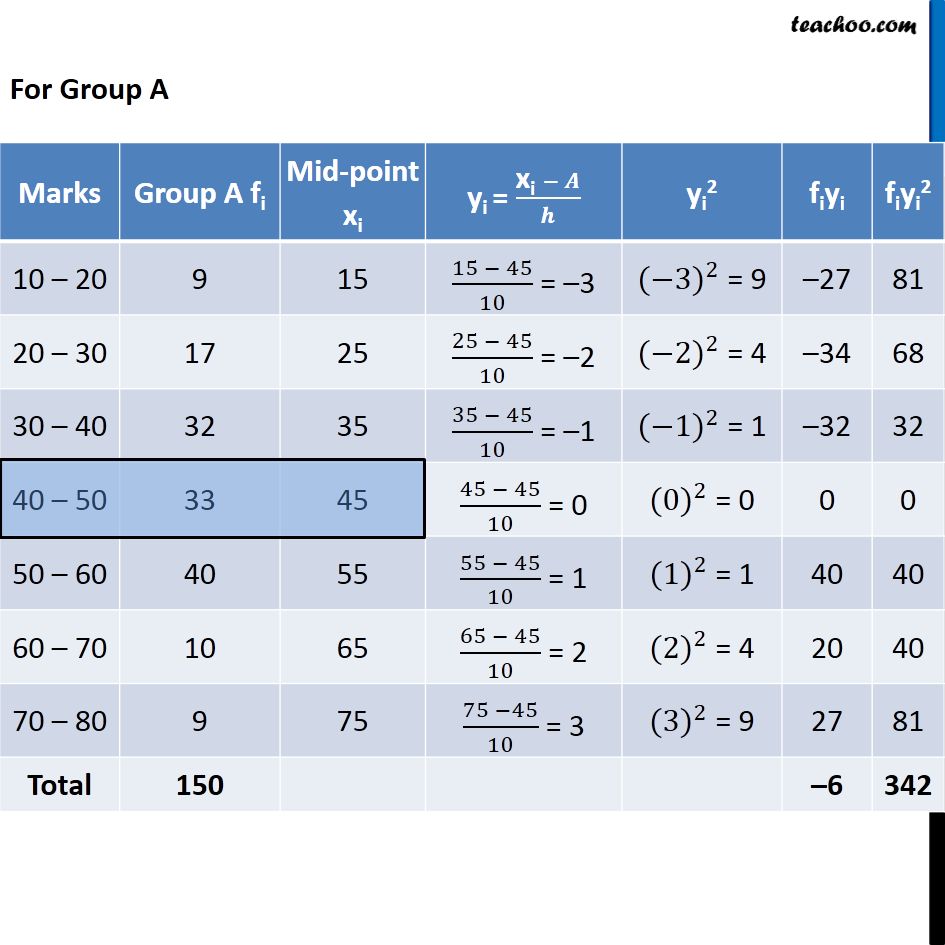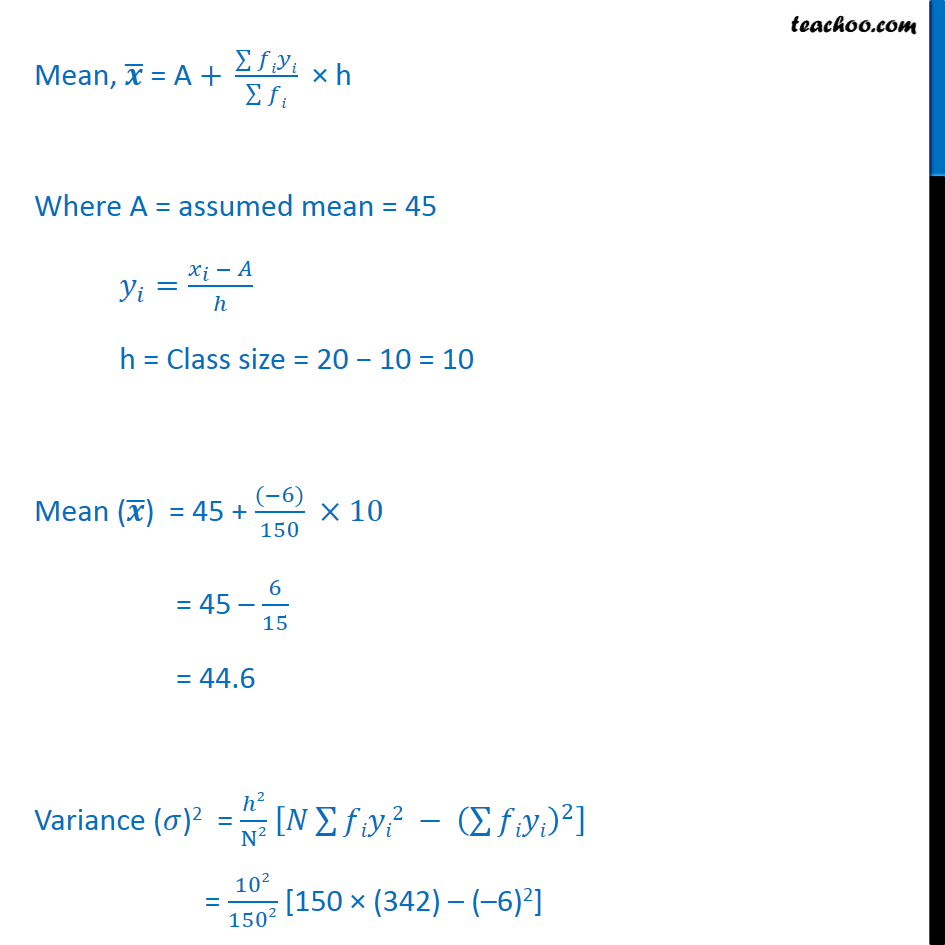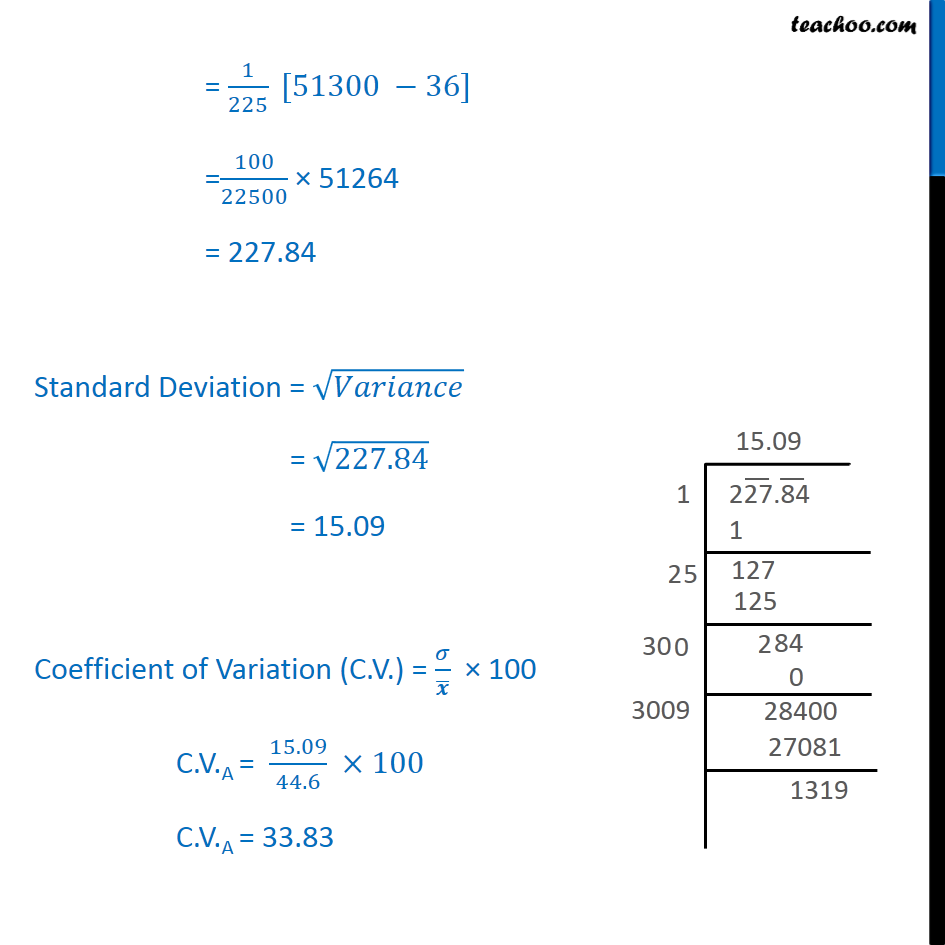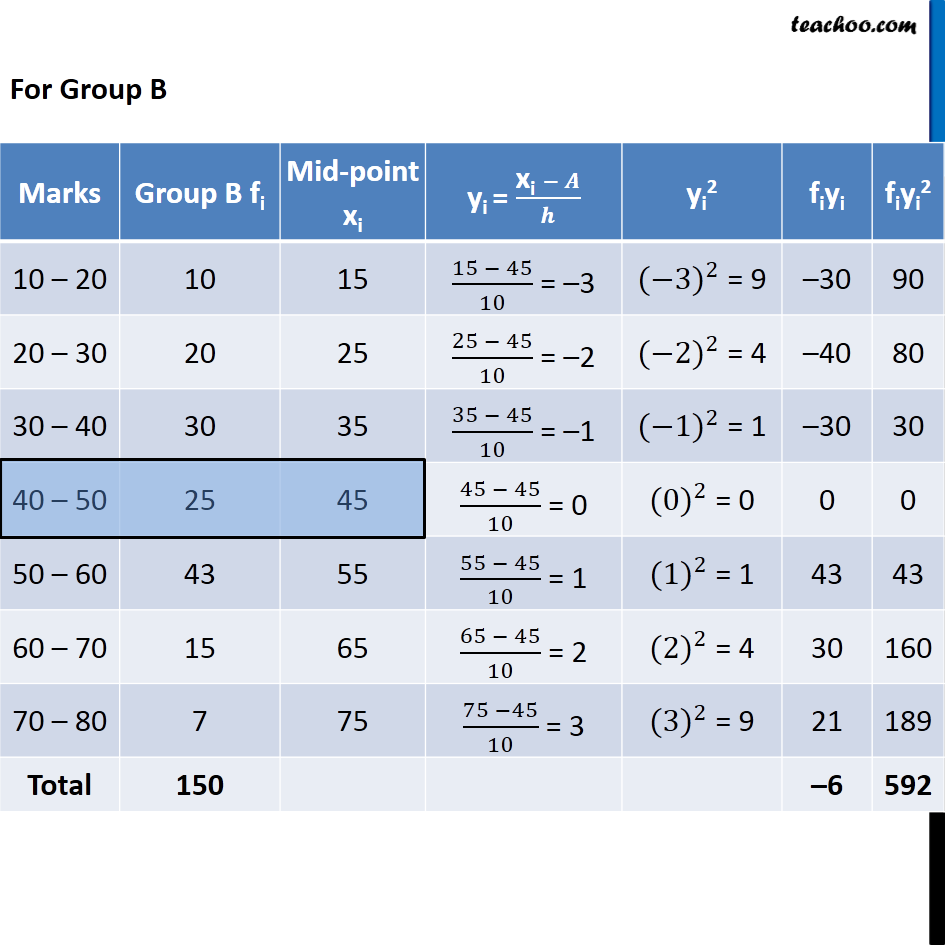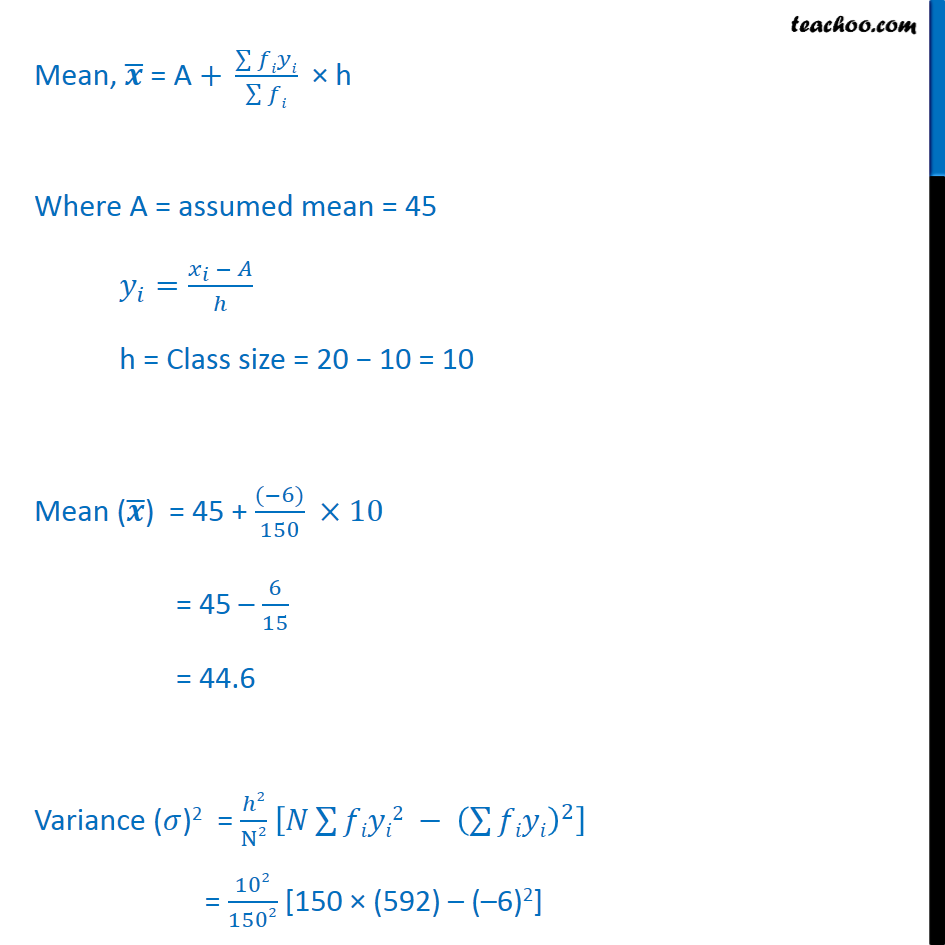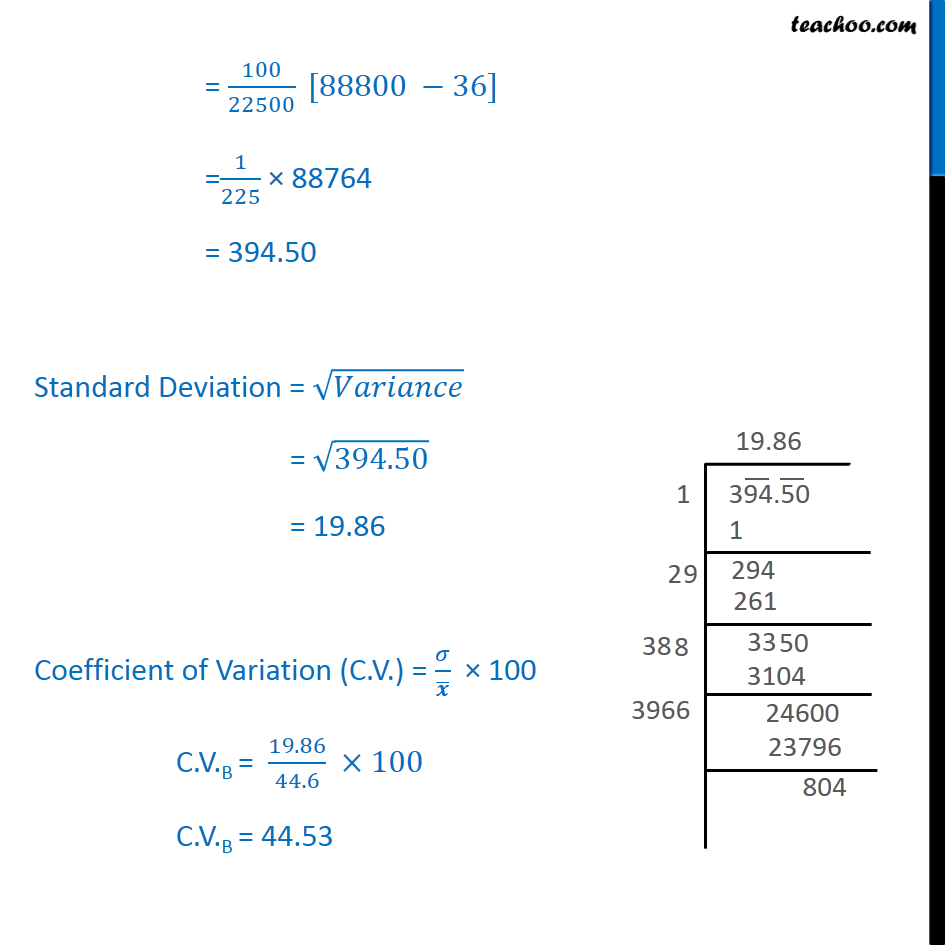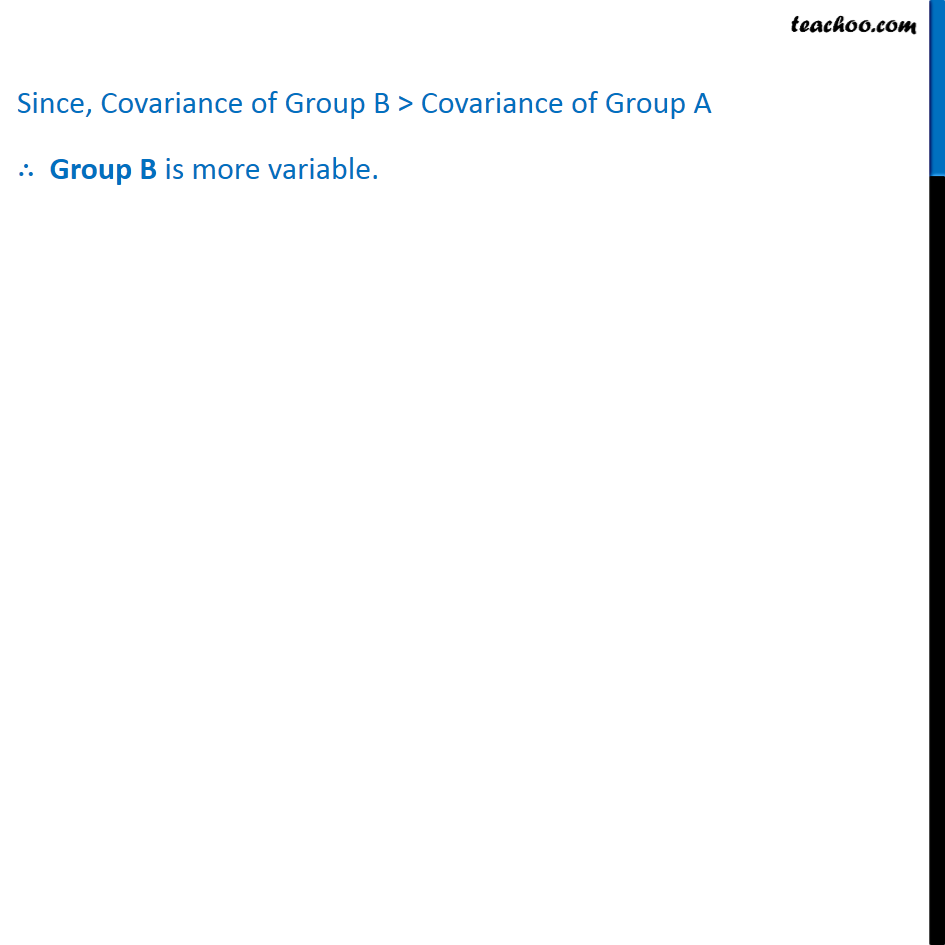1. Chapter 15 Class 11 Statistics (Term 1)
2. Serial order wise
3. Ex 15.3

Transcript

Ex 15.3, 1 From the data given below state which group is more variable, A or B? The group having more Coefficient of Variation will be more variable. Coefficient of Variation (C.V.) = 𝜎/𝒙 ̅ × 100 where 𝜎 = Standard Deviation 𝒙 ̅ = Mean Finding standard deviation & mean of both Group A and Group B.

Ex 15.3